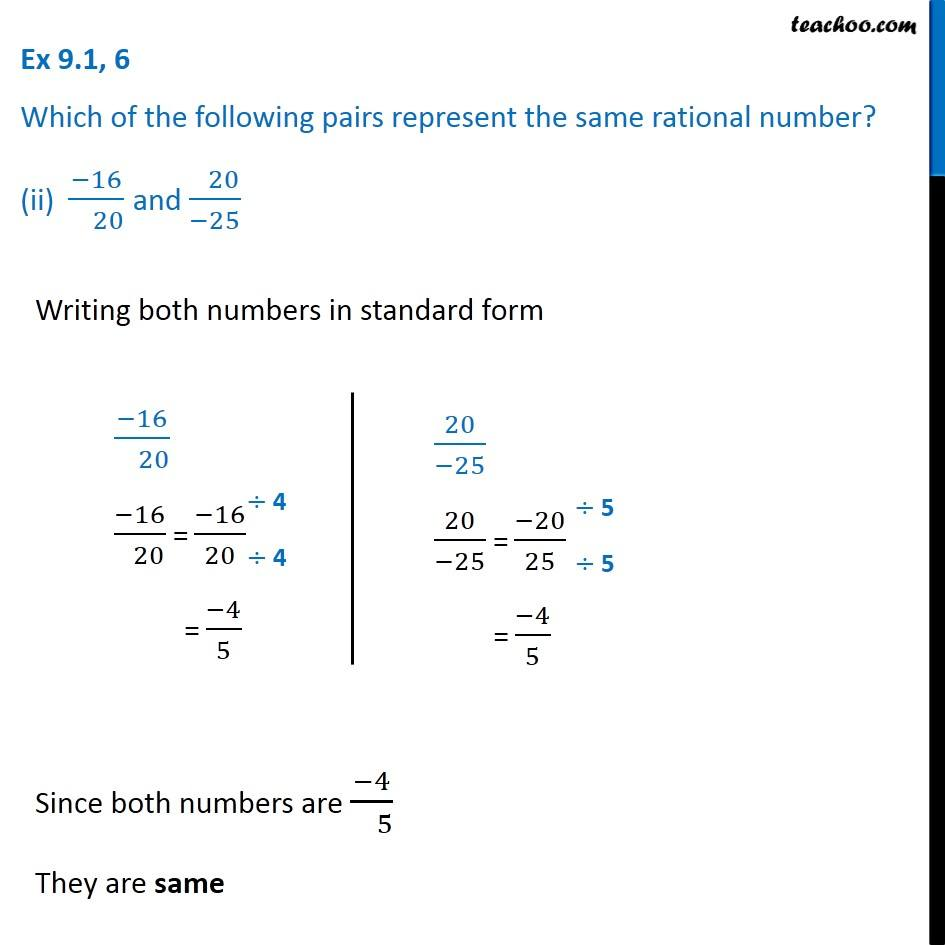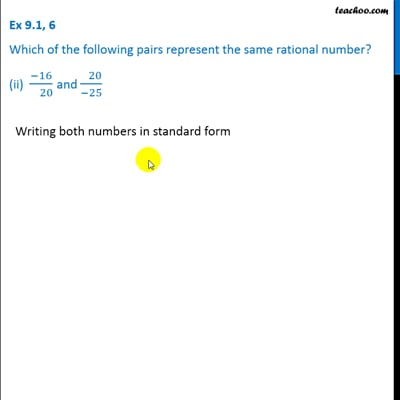Ex 9.1

Chapter 9 Class 7 Rational Numbers
Serial order wiseThis video is only available for Teachoo black users

Get live Maths 1-on-1 Classs - Class 6 to 12

### Transcript

Ex 9.1, 6 Which of the following pairs represent the same rational number? (ii) (−16)/( 20) and ( 20)/(−25) Writing both numbers in standard form (−16)/( 20) (−16)/( 20) = (−16)/20 = (−4)/5 20/(−25) 20/(−25) = (−20)/25 = (−4)/5 Since both numbers are (−4)/( 5) They are same